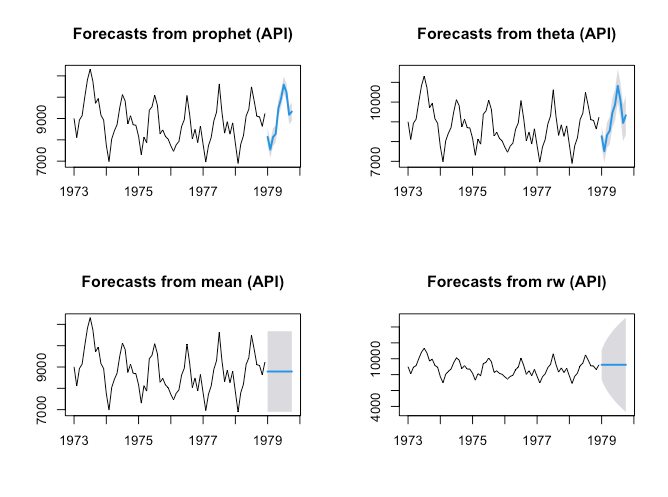Want to share your content on R-bloggers? click here if you have a blog, or here if you don't.

A few weeks ago, I introduced a forecasting API (Application Programming Interface). The application can be found here:

https://techtonique2.herokuapp.com/

So far, as of 2022-11-23, this API contains four methods for univariate time series forecasting (with prediction intervals):

• `mean` a (not so naïve) benchmark method, whose prediction is the sample mean.
• `rw` a (not so naïve) benchmark method, whose prediction is the last value of the input time series.
• `theta` is the forecasting method described in  and , which won the M3 competition.
• `prophet` is a popular model described in .

In this post, I’ll present two packages, one implemented in R and one in Python, which are designed for smoothing users’ interaction with the API. You can create similar high-level packages in other programming languages, by using this tool and this page.

Content

• 0 – Install packages in R or Python
• 1 – Create an account with `create_account`
• 2 – Get a token for authentication using `get_token`
• 3 – Requests for forecasts with `get_forecast`

## 0 – Install packages in R or Python:

• In Python
```pip install forecastingapi
```
• In R
```library(devtools)
devtools::install_github("Techtonique/forecastingapi/R-package")
library(forecastingAPI)
```

## 1 – Create an account with `create_account`:

• In Python
```import forecastingapi as fapi

print(res_create_account)
```
• In R
```forecastingAPI::create_account(username = "[email protected]", password = "pwd") # choose a better password
```

## 2 – Get a token for authentication using `get_token`

• In Python
```token = fapi.get_token(username = "[email protected]", password = "pwd")
print(token)
```
• In R
```token <- forecastingAPI::get_token(username = "[email protected]", password = "pwd")
```

The token is valid for 5 minutes. After 5 minutes, it must be renewed, using `get_token`.

## 3 - Requests for forecasts with `get_forecast`:

• In Python
```path_to_file = '/Users/t/Documents/datasets/time_series/univariate/USAccDeaths.csv' # (examples:https://github.com/Techtonique/datasets/tree/main/time_series/univariate)

res_get_forecast = fapi.get_forecast(file=path_to_file, token=token)

print(res_get_forecast)

res_get_forecast2 = fapi.get_forecast(file=path_to_file,
token=token, start_training = 2, n_training = 7, h = 4, level = 90)

print(res_get_forecast2)

res_get_forecast3 = fapi.get_forecast(file=path_to_file,
token=token, date_formatting="ms",
start_training = 2, n_training = 7, h = 4, level = 90)

print(res_get_forecast3)

res_get_forecast4 = fapi.get_forecast(file=path_to_file,
token=token, method = "prophet")

print(res_get_forecast4)
```
• In R
```path_to_file <- '/Users/t/Documents/datasets/time_series/univariate/USAccDeaths.csv' # (examples:https://github.com/Techtonique/datasets/tree/main/time_series/univariate)

f_theta <- forecastingAPI::get_forecast(file = path_to_file, token = token,
method = "theta", h=10, level = 95)

f_mean <- forecastingAPI::get_forecast(file = path_to_file, token = token,
method = "mean", h=10, level = 95)

f_rw <- forecastingAPI::get_forecast(file = path_to_file, token = token,
method = "rw", h=10, level = 95)

f_prophet <- forecastingAPI::get_forecast(file = path_to_file, token = token,
method = "prophet", h=10, level = 95)

```Assimakopoulos, V., & Nikolopoulos, K. (2000). The theta model: a decomposition approach to forecasting. International journal of forecasting, 16(4), 521-530.

 Hyndman, R. J., & Billah, B. (2003). Unmasking the Theta method. International Journal of Forecasting, 19(2), 287-290.

 Taylor, S. J., & Letham, B. (2018). Forecasting at scale. The American Statistician, 72(1), 37-45.